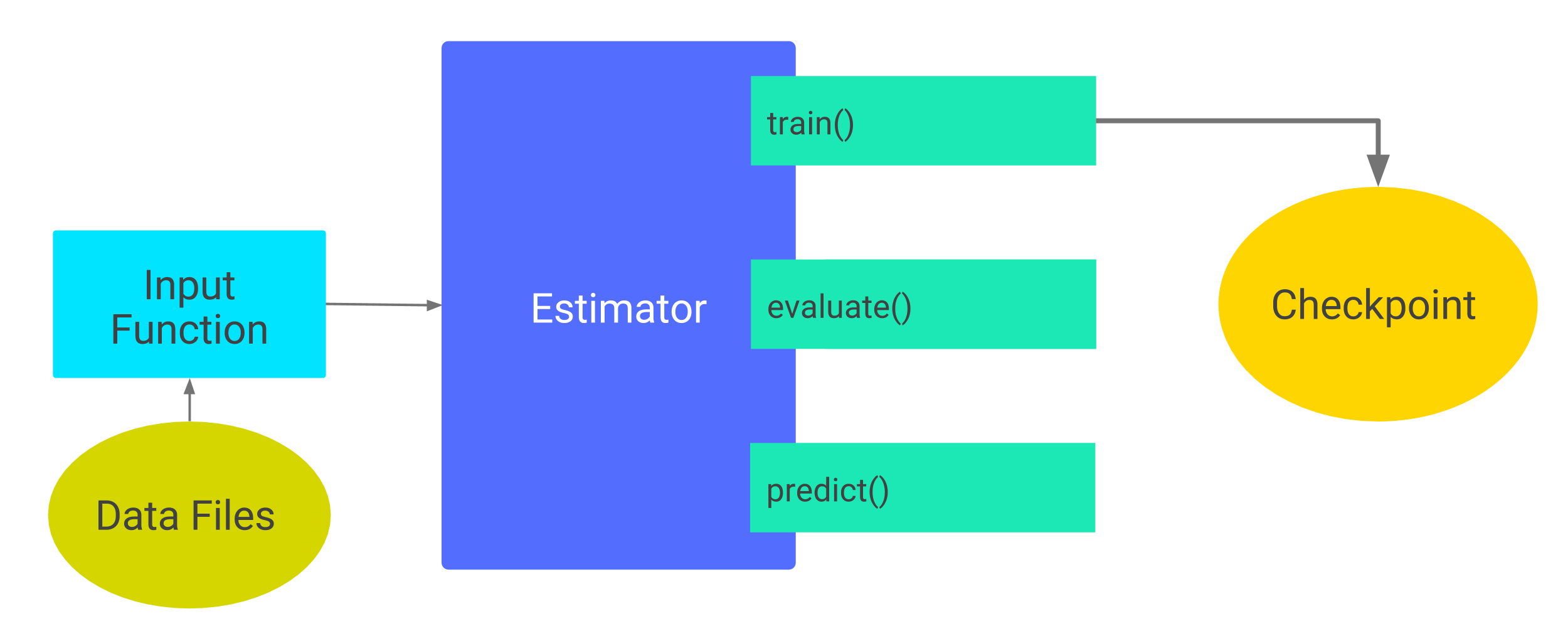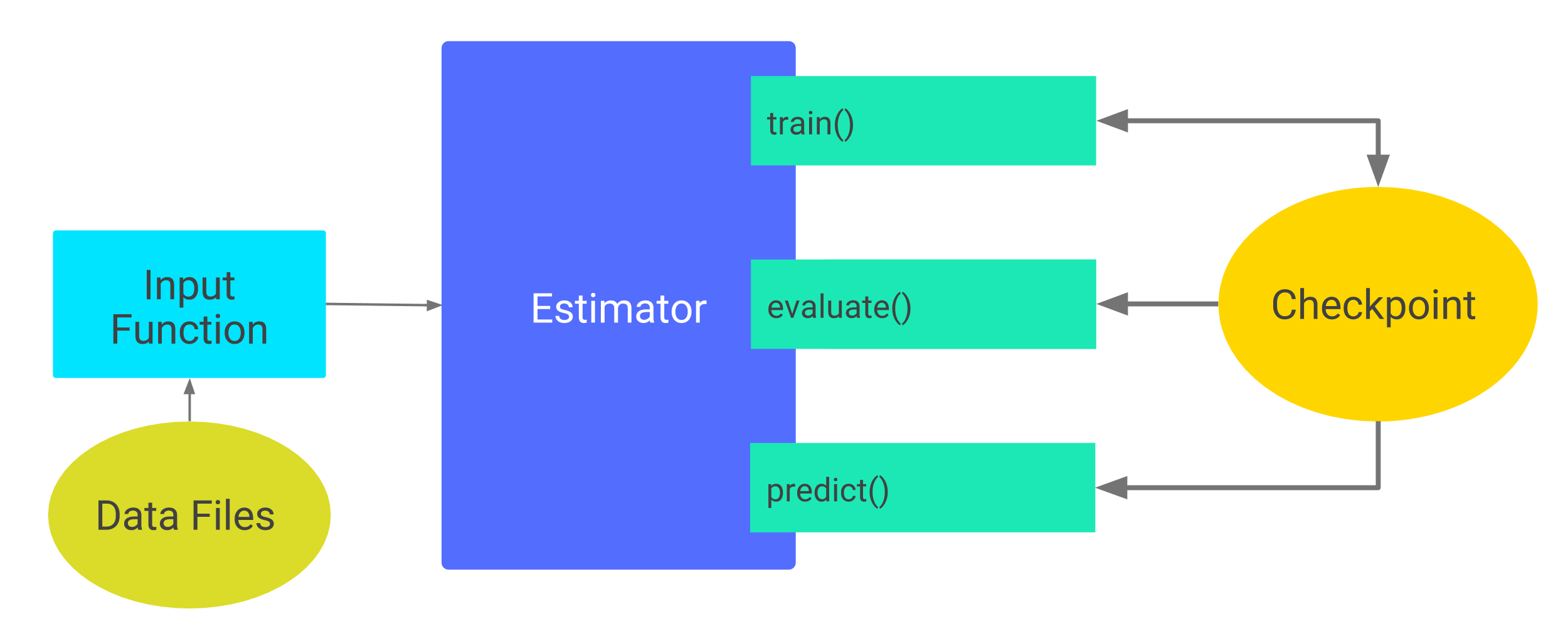# 检查点

•   检查点（checkpoints）：这是一种依赖于创建模型代码的格式。
•   SavedModel：这是一种与创建模型代码无关的格式。

## 示例代码

``````git clone https://github.com/tensorflow/models/
cd models/samples/core/get_started
``````

## 保存未训练完的模型

Estimators 自动将下列内容写到磁盘上：

•   检查点：训练过程中生成的不同版本的模型。
•   事件文件：包含一些用于 TensorBoard 可视化的信息

``````classifier = tf.estimator.DNNClassifier(
feature_columns=my_feature_columns,
hidden_units=[10, 10],
n_classes=3,
model_dir='models/iris')
``````

``````classifier.train(
input_fn=lambda:train_input_fn(train_x, train_y, batch_size=100),
steps=200)
````````````\$ ls -1 models/iris
checkpoint
events.out.tfevents.timestamp.hostname
graph.pbtxt
model.ckpt-1.data-00000-of-00001
model.ckpt-1.index
model.ckpt-1.meta
model.ckpt-200.data-00000-of-00001
model.ckpt-200.index
model.ckpt-200.meta
``````

### 默认检查点目录

``````classifier = tf.estimator.DNNClassifier(
feature_columns=my_feature_columns,
hidden_units=[10, 10],
n_classes=3)

print(classifier.model_dir)
``````

`tempfile.mkdtemp` 函数会为你在操作系统中选择一个安全的临时目录。比如，在 macOS 操作系统中，一个典型的临时目录为：

``````/var/folders/0s/5q9kfzfj3gx2knj0vj8p68yc00dhcr/T/tmpYm1Rwa
``````

### 检查点的保存频率

•   每隔 10 分钟保存一个检查点（即 600 秒）。
•   当 `train` 方法开始执行（即第一次循环）和执行结束（最后一次循环）时，会各保存一个检查点。
•   保留目录中最近 5 个检查点。

1. `tf.estimator.RunConfig`
2. 当实例化 Estimator 时，将此 `RunConfig` 对象传递给 Estimator 的 `config` 参数。

``````my_checkpointing_config = tf.estimator.RunConfig(
save_checkpoints_secs = 20*60,  # Save checkpoints every 20 minutes.
keep_checkpoint_max = 10,       # Retain the 10 most recent checkpoints.
)

classifier = tf.estimator.DNNClassifier(
feature_columns=my_feature_columns,
hidden_units=[10, 10],
n_classes=3,
model_dir='models/iris',
config=my_checkpointing_config)
``````

## 恢复你的模型

1. 创建定制化 Estimator
2. 这个 Estimator 从最近的检查点中恢复出数据，用于初始化新模型的权重值。### 避免不好的恢复

``````classifier = tf.estimator.DNNClassifier(
feature_columns=feature_columns,
hidden_units=[10, 10],
n_classes=3,
model_dir='models/iris')

classifier.train(
input_fn=lambda:train_input_fn(train_x, train_y, batch_size=100),
steps=200)
``````

``````classifier2 = tf.estimator.DNNClassifier(
feature_columns=my_feature_columns,
hidden_units=[20, 20],  # 修改模型中的神经元个数
n_classes=3,
model_dir='models/iris')

classifier.train(
input_fn=lambda:train_input_fn(train_x, train_y, batch_size=100),
steps=200)
``````

``````...
InvalidArgumentError (see above for traceback): tensor_name =